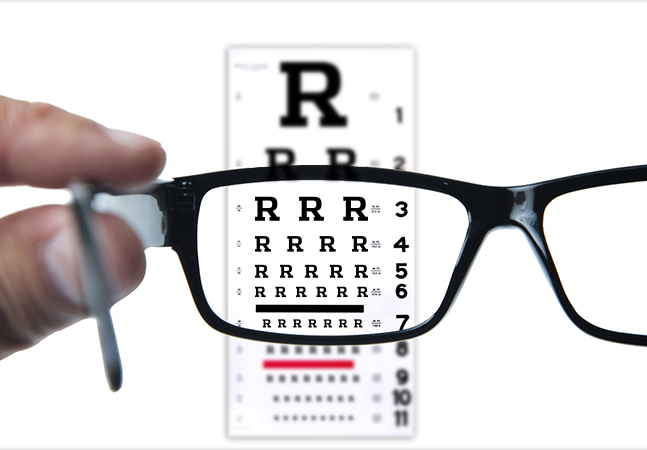#### The Data Science Lab### Data Clustering Using R

Find the patterns in your data sets using these Clustering.R script tricks.### R Language OOP Using S3

The S3 OOP model is still widely used, so let's use write S3-style OOP code via the R language.### Logistic Regression Using R

I predict you'll find this logistic regression example with R to be helpful for gleaning useful information from common binary classification problems.### Neural Networks Using the R nnet Package

The R language simplifies the creation of neural network classifiers with an add-on that lays all the groundwork.### Results Are in -- the Sign Test Using R

The R language can be used to perform a sign test, which is handy for comparing "before and after" data.### R Language Searching and Sorting

A language that's data-intensive naturally should have a way to dig into the data effectively. Here's a look at some of the R functions for searching and sorting through it all.### R Language Basic Data Structures

Vectors, lists, arrays, matrices and data frames -- a look at five of the most fundamental data structures built into R.### Permutations Using R

R has limited support for mathematical permutations, but it's there. Here's what R is capable of accomplishing.### How the R Language Does OOP

It's not quite like C# or Python, but the R language's object-oriented programming capabilities are getting better with each iteration. Let's take a look at what .NET developers are able do now with OOP in R6.### Classic Stats, Or What ANOVA with R Is All About

New to this type of analysis? It's a classic statistics technique that is still useful. Here's a technique for doing a one-way ANOVA using R.### Program-Defined Functions in R

The three most common open source technologies for writing data science programs are Python, SciLab, and R. Here's how to write program-defined functions in R.### Chi-Square Tests Using R

R is the perfect language for creating a variety of chi-square tests, which are used to perform statistical analyses of counts of data. Here's how, with some sample code.### Fundamentals of T-Test Using R

Linear regression was easy, right? Now, let's check out t-test analysis using R.### Linear Regression with R

Now that you've got a good sense of how to "speak" R, let's use it with linear regression to make distinctive predictions.### Introduction to R for .NET Developers

C# developers who want to wring more meaningful info from large sets of data should get cozy with the statistical computing language known as R. Let's get familiar with R in this new series.

Upcoming Events